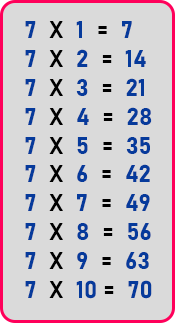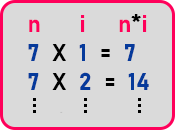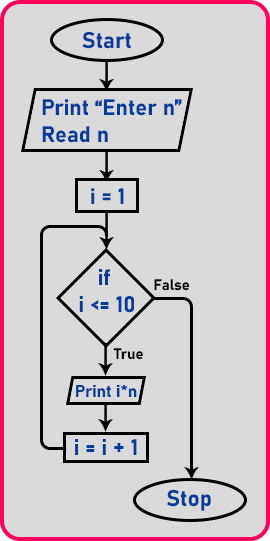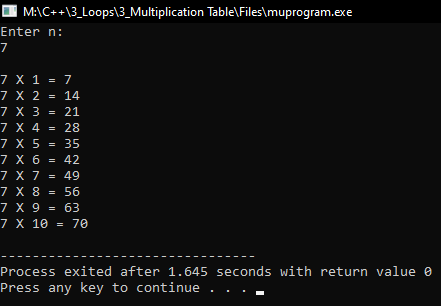# Multiplication Table for a Given Number in C++

## Multiplication Table for a Given Number in C++

In this article, I am going to discuss the Multiplication Table for a Given Number in C++ with Examples. Please read our previous articles, where we discussed For loop in C++ with Examples.

##### Multiplication Table for a Given Number in C++:

Let us write a program for displaying a multiplication table for a given number. This problem uses a loop. Whenever you have repeating statements. So, the first task or challenge for a student will be to identify whether the procedure is having repeating statements or not. Let us learn how to understand the procedure and how to pick a loop and solve a problem. Let us see a multiplication table.We have an example of the multiplication table. Here we have written the multiplication table of ‘7’ up to 10. We want to print the multiplication table in the same style that we have written above. Now if you observe, every time ‘7’ is multiplied by something and we get the result and displayed, then again get multiplied by some other number and get the result and so on.

Here we are printing the multiples of ‘7’ up to 10 times. Then in this what are the thing we should know? First, we should know the number which we want the multiples like in this case, the number was ‘7’. Second, we should know where we want the multiple, in this case, we print up to 10. Then how we are getting this result?We should take ‘n’ and ‘i’ and multiple both and get the result ‘i*n’. In every step, ‘i’ should be increased and number ‘n’ same. So, this is how we framed a procedure for this. So, the procedure is that we multiply ‘i’ and ‘n’, increase the value of ‘i’ in every step and ‘n’ remains the same. Now let us show you the flowchart for this problem.

##### Multiplication Table Flowchart:

Let us look at the flow chart.First, we take a number from the user. Then we should initialize the counter to ‘1’. Initially, it should be 1. Then how many times we should print the multiples? 10 is sufficient. So when ‘i’ will reach ‘10’ then it should stop here. So, we will give the condition as ‘if (i <= 10)’. If the condition will true then print the result of multiplication ‘i*n’. And after printing, increment the ‘i’ as ‘i = i + 1’. As you can see in the flowchart, it will again check the condition, then print the result, the increment ‘i’, and so on until the condition becomes false. If the condition will false, then simply stop the loop. Now let us look at the program.

##### Program to print Multiplication Table for a given number in C++:
```#include <iostream>
using namespace std;
int main()
{
int n;
cout << "Enter n:" << endl;
cin >> n;
cout << endl;

for (int i = 1; i <= 10; i++)
{
cout << n << " X " << i << " = " << i * n << endl;
}

return 0;
}
```
###### Output: